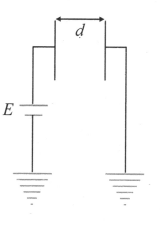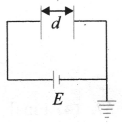Kirchhoff's rules for capacitors
Question

# Two plates, each of area A, are placed parallel to each other at a distance d. One plate is connected to a battery of emf E and its negative is earthed. The other plate is also earthed. The charge drawn by plate isModerate
Solution

## The circuit can be redrawn as shown in figure. Charge on capacitor is$Q=CE=\frac{{\epsilon }_{0}AE}{d}$Options 1 and 3 and 4 are incorrect.

Get Instant Solutions